## Deep Learning with Keras and TensorFlow

Certification Training
3869 Learners
9 Chapters +

# Recurrent Neural Networks Tutorial

Welcome to the eighth lesson, ‘Recurrent Neural Networks’ of the Deep Learning Tutorial, which is a part of the Deep Learning (with TensorFlow) Certification Course offered by Simplilearn. This lesson focuses on Recurrent Neural Networks along with time series predictions, training for Long Short-Term Memory (LSTM) and deep RNNs.

Let us begin with the objectives of this lesson.

## Objectives

After completing this lesson on Recurrent Neural Networks, you’ll be able to:

• Explore the meaning of Recurrent Neural Networks (RNN)

• Understand the working of recurrent neurons and their layers

• Interpret how memory cells of recurrent neurons interact

• Implement RNN in TensorFlow

• Demonstrate variable length input and output sequences

• Explore how to train recurrent neural networks

• Review time series predictions

• Describe training for Long Short-Term Memory (LSTM) and Deep RNNs

• Analyze word embeddings

Let us understand sequence prediction in the next section.

## Sequence Prediction

You predict based on the sequence or past experience all the time.

A fielder tries to predict where the cricket ball will land

Other examples include stock market predictions, an autonomous car trying to decide car trajectory to stay safe, or predicting words in a sentence.

In the next section, let us study sequence prediction in deep learning.

Are you curious to know what Deep Learning is all about? Watch our Course Preview here!

## Sequence Prediction in Deep Learning

Feedforward networks are widely used for image classification and statistical analysis. In these networks, connections between the units do not form a reverse circular loop.

As the name suggests, information moves in one direction—from input nodes, through hidden nodes, to the output nodes.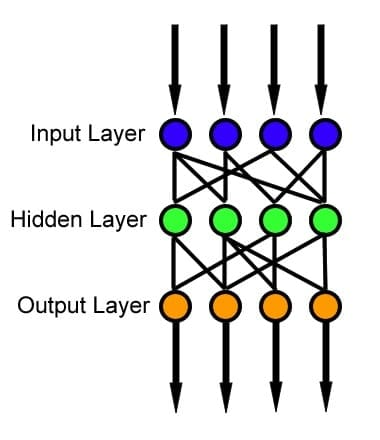The limitation of feedforward networks is that the output of the complete network does not directly depend on the previous output of the same network. This means that the concept of "recurrence" is missing. They have separate parameters for each input feature.

Thus the sequence of outputs are independent of each other, which might not be suitable for predictions of words in a sentence. Hence, Recurrent Neural Networks (RNN) prove to be a better choice for sequence prediction than feedforward networks.

In the next section, let us study the recurrent neural networks.

## Recurrent Neural Networks: Definition

Recurrent neural networks are a class of artificial neural networks that create cycles in the network graph in order to exhibit dynamic temporal behavior. In simple words, RNNs involve recurrent or circular loops between the neurons, where the output of the network is fed back as an additional input to the network for subsequent processing.

In the next section, let us focus on the need for recurrent neural networks.

## Need for Recurrent Neural Networks

Recurrent Neural Networks can process sequences of large size as well as those with variable length. It is based on the sharing of parameters across different parts of the model. Parameters share the same weights across several time steps.

Each member of the output is a function of the previous member’s output. It is produced using the same update rule that is applied to all previous outputs. This recurrence imparts a memory to the network topology.

In the next section, let us discuss the use cases of the recurrent neural network.

## Use Cases of Recurrent Neural Networks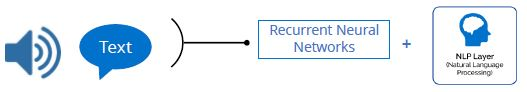Some of the remaining use cases are listed below:

• RNNs can generate sentences, image captions, or even notes of a melody.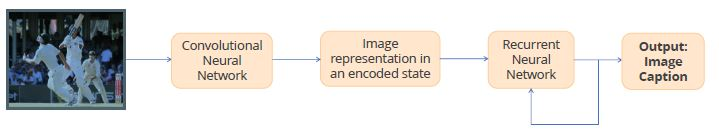• Anticipating car trajectories to avoid accidents in autonomous cars

• Analyzing time series data such as stock prices, and suggesting to brokers when to buy or sell stocks

• Enabling handwriting and speech recognition

• Google’s Magenta project lets you create art and music using RNN algorithms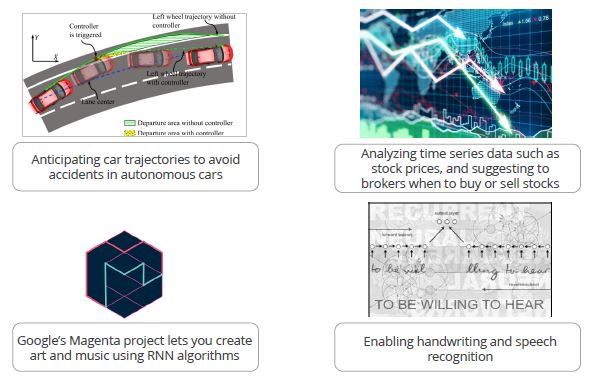In the next section, let us focus on the layers of recurrent neurons.

## Recurrent Neurons

An RNN looks like a feedforward network except it has connections pointing backward. This tiny network can be plotted against the time axis as shown in the figure.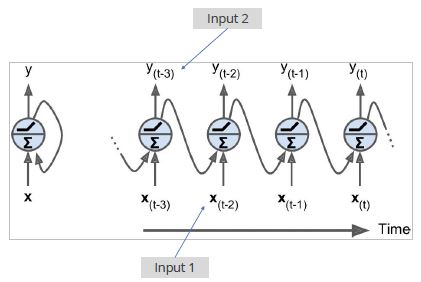The figure shows one neuron that receives inputs, produces an output and sends that output back to itself. This is called unrolling the network through time.

In this diagram:

t represents a time step also called frame.

The recurrent neuron receives two inputs:

• Input 1: Input 1 is the actual input to the network at a particular time step. It is represented by xt at each increment of t.

• Input 2: Input 2 is the output of the previous time step fed as an additional input to the current time step. It is represented by yt-1.

## The Layer of Recurrent Neurons

Using the recurrent neurons, you can create a layer of recurrent neurons. Once unrolled, it looks like the image on the right. In the single recurrent neuron shown before, the input x was a vector and output y was a scalar.Here, in a layer of recurrent neurons, both x and y are vectors.

Let us study adding weights to recurrent neurons in the next section.

## Adding Weights to Recurrent Neurons

Each recurrent neuron has two sets of weights, one for the input xt and the other for the outputs of the previous time step, yt-1.

Let’s call these weight vectors wx and wy.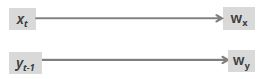All the weight vectors can be placed into two weight matrices if the entire recurrent layer is considered instead of one recurrent neuron.

These weight matrices can be represented as follows:

Wx

Wy

## Computing Output for a Single Input

The output vector of the recurrent layer containing a single neuron can be computed as follows: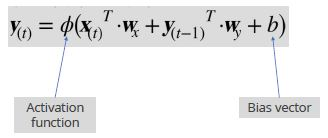## Computing Output for Mini-batch

A recurrent layer’s output can be computed for a whole mini-batch of data samples by placing all the inputs at time step t in an input matrix Xt.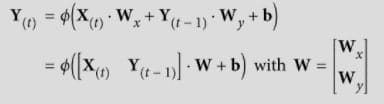The terms in the equation are discussed below: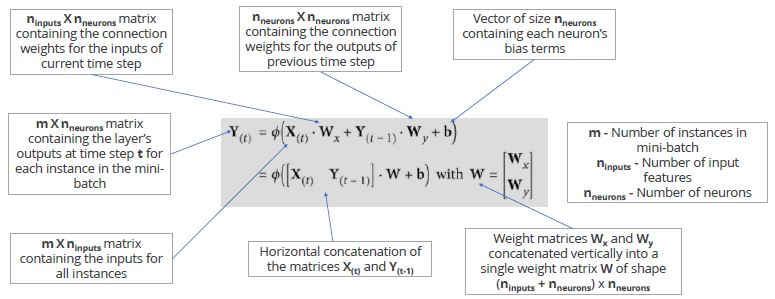In the next section, let us discuss the memory cells of recurrent neurons.

## Memory Cells of Recurrent Neurons

A recurrent neuron is called a memory cell owing to the fact that this neuron tends to preserve memory across multiple time steps. A recurrent neuron has memory. This is evident from the fact that its output at time step t is a function of all inputs from previous time steps.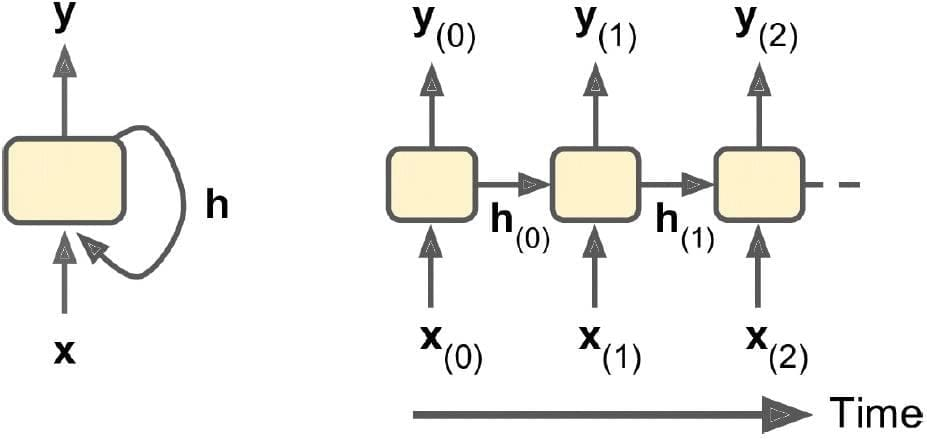The part of a neural network that preserves some state across time steps is referred to as a memory cell.

## Hidden State of Memory Cells

A cell’s hidden state can be different from its output as shown in the image. y(t)is different than h(t). At other times, y(t) and h(t)can be the same.

A cell’s state at time step t, denoted by h(t), is a function of some inputs at that time step and its state at the previous time step:

h(t)= f(h(t-1), x(t))

The output y at time step t is also a function of all previous states and the current input.

In the next section, let us focus on the various configurations of RNN.

## Various Configurations of Recurrent Neural Networks

RNNs can be arranged in multiple configurations to serve various purposes. The four configurations are described as follows:

Sequence to Sequence

Here, you feed a sequence of inputs to the RNN, and you get a sequence of outputs. For example, RNN is used for time series predictions such as stock price.

In this case, the input is stock price pattern until step and output is a time step shifted into the future.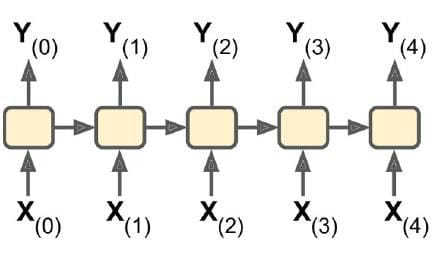Sequence to Vector

Here, you take a sequence of inputs and generate just a single output; for example, sentiment score (+1 or -1) for a movie review.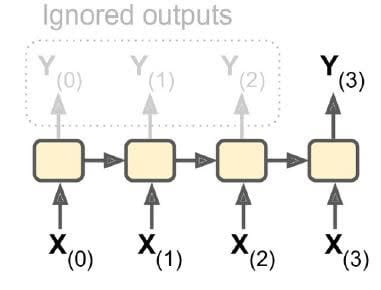Vector to Sequence

Here, the RNN takes one input and produces a sequence of outputs; for example, producing a caption for an image.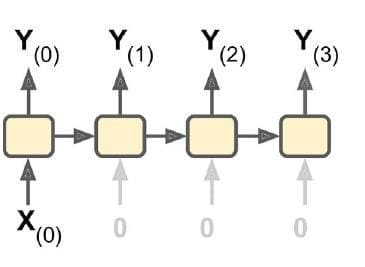Sequence to Sequence

This type has two networks embedded together.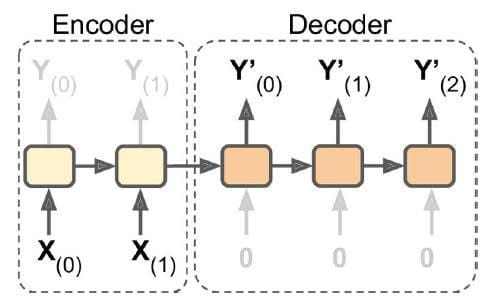The first RNN network takes a sequence of inputs to produce an output vector.

This output vector is fed to the second RNN that produces a sequence of outputs.

The first box in the diagram that is Encoder is the sequence to vector and Decoder is the vector to sequence; for example, language translation.

To summarize, here is a high-level view of various RNN configurations: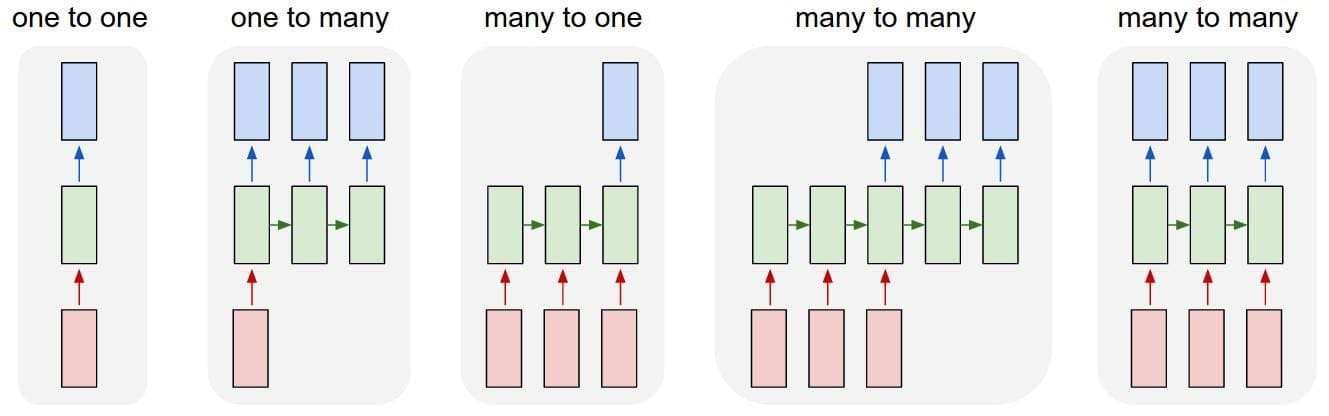Rectangles –Represent vectors

Arrows –Represent functions

Red Rectangles–Input vectors

Blue Rectangles –Output vectors

Green Rectangles –RNN’s state

One to one -

Vanilla mode of processing without RNN, from fixed-sized input to fixed-sized output

For example Image classification

One to many -

Sequence output

For example, Image captioning takes an image and outputs a sentence of words

Many to one -

Sequence input

For example Sentiment analysis, where a given sentence is classified as expressing a positive or negative sentiment

Many to many -

Sequence input and sequence output

For example Machine translation, where an RNN reads a sentence in English and then outputs a sentence in French

Many to many -

Synced sequence input and output

For example Video classification, where you wish to label each frame of the video

Let us discuss the RNN in TensorFlow in the next section.

## Develop a Simple Recurrent Neural Networks Model

The code given below builds a basic RNN (without using RNN operations of TensorFlow) with two-time steps.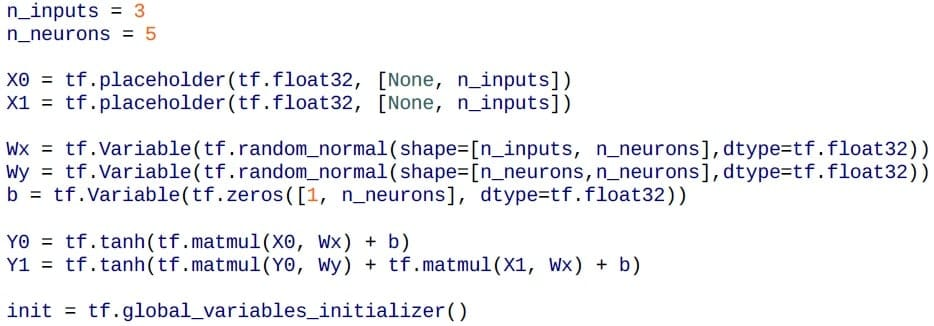At each time step, there are five recurrent neurons and the input is a vector with three units.

You use tanh activation function at each time step.

The network looks like a regular two-layer feedforward network with the following differences:

• Weights are shared by both layers

• You feed inputs at each layer and get output at each layer

Let’s feed a mini-batch of inputs, which consists of the input vectors at both time steps.

Each input would have four data instances and you get outputs at both time steps.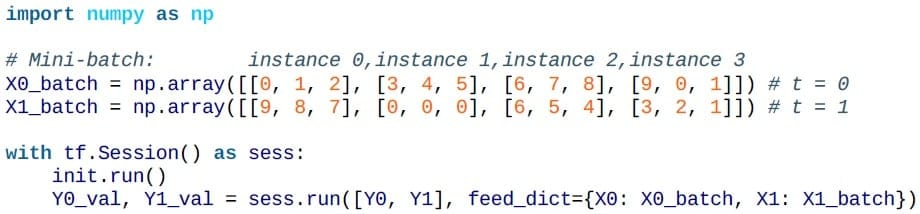The output contains vectors for entire mini-batch for all samples.

Since n_neurons per layer was five, you get an output vector containing five output values per data instance.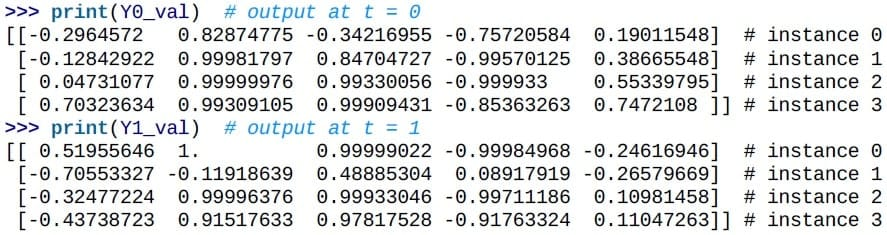For the larger number of time steps like 100 steps, the graph gets very big. This can be simplified by using TensorFlow RNN operations.

## Basic Recurrent Neural Networks Cell

A Basic RNN Cell in TensorFlow is the basic RNN unit which when unrolled creates copies of the cell for many time steps.

## Implement Basic Recurrent Neural Networks Cell with TensorFlow

The function static_rnn creates an unrolled network by chaining basic cells as shown in the code given below.

The static_rnn function returns:

• output_seq –List of output tensors for every time step

• states–Tensor containing final states of the network

For a basic cell, a state is the same as the output of the previous time step. For example, yi=hi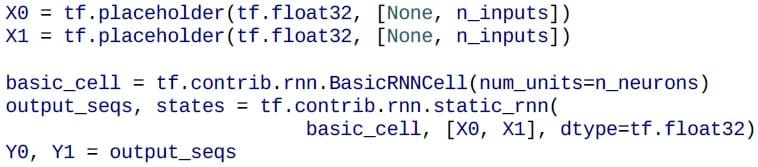The following more efficient code defines the input of shape [None, n_steps, n_inputs], where the first parameter is mini-batch size.

The unstack command extracts input sequences for each time step, that is, X_seqs which has shape [None, n_inputs].

transpose command is used to swap the first two dimensions, that is, n_steps and mini-batch size.

The rest of the code stays the same as before.

Final line here stacks the output tensors into a single tensor of shape [None, n_steps, n_inputs].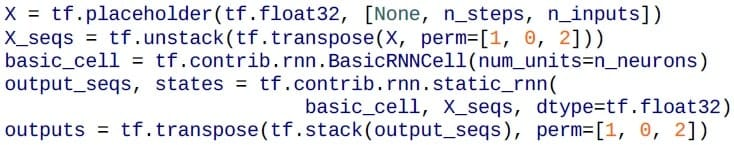As evident in the following code, you feed a mini-batch as before.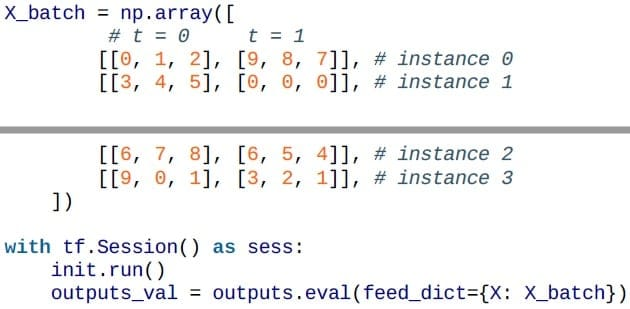In the next section, let us focus on the dynamic unrolling to simplify the logic and avoid OOM errors.

## Dynamic Unrolling to Simplify the Logic and Avoid OOM Errors

The output of the code is: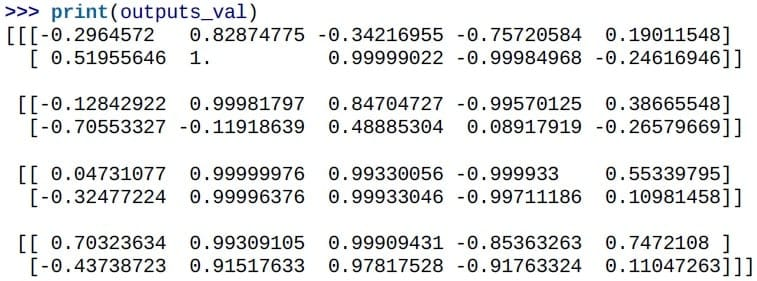So far you were still building one cell per time step. The graph can turn out to be complex for 50-time steps.

This can lead to Out of Memory (OOM) errors during backpropagation since the memory must store all output tensors in feedforward pass to calculate gradients during the reverse pass.

This is solved by dymanic_rnn function.

The dynamic_rnn function accepts a tensor for all inputs at every time step of shape [None, n_steps, n_inputs], and it outputs a single tensor for all outputs at each time step of shape [None, n_steps, n_inputs].

There is no need to stack, unstack, or transpose.

The following code is cleaner and creates the same network as before.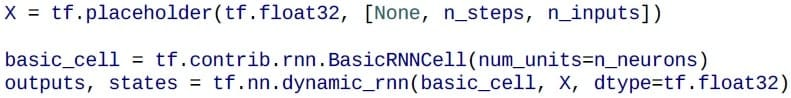In the next section, let us discuss handling sequences of variable length.

## Handling Sequences of Variable Lengths

Let us discuss how to handle input and sequences of variable lengths below:

Variable Length Input Sequences

Instead of the fixed-sized input sequence, one could have variable length input sequence. The length of the input sequence is provided by sequence_lengthparameter.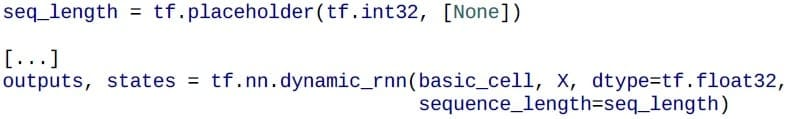If the length of one input sequence is shorter than others, you can zero-pad it so that it fits the input tensor. (Zero padding means filling up with zeros to make the length of the elements consistent with those of the other elements).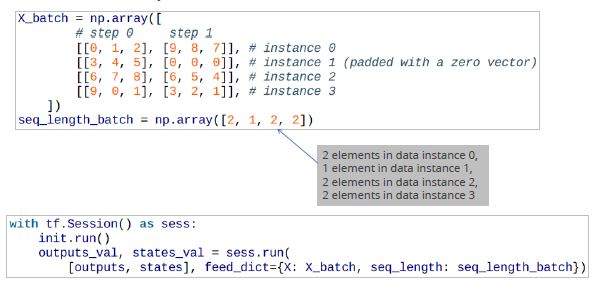The output tensor is shown in the output below. The outputs are zero vector if the input sequence is zero input.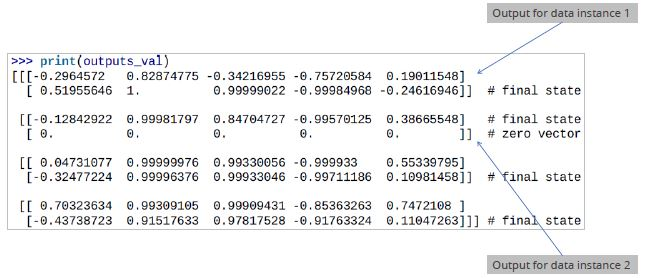The statestensor (which contains final state of each cell) looks as follows: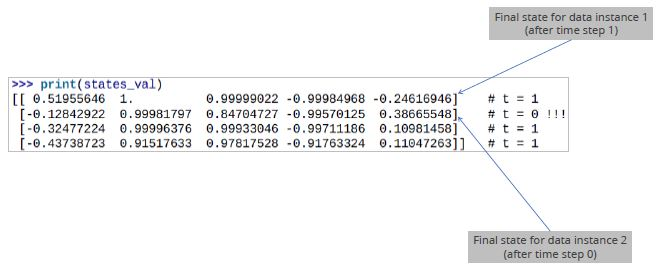Variable Length Output Sequences

The sequence_length parameter can be set as before if the output sequence has the same length as the input sequence.

If output length sequence varies as in language translation model, you can define a special output called an end-of-sequence token (EOS token), which will mark the end of the output sequence.

In the next section, let us learn about training recurrent neural networks.## Backpropagation through Time

To train an RNN, unroll it through time and then apply backpropagation. This is called backpropagation through time (BPTT).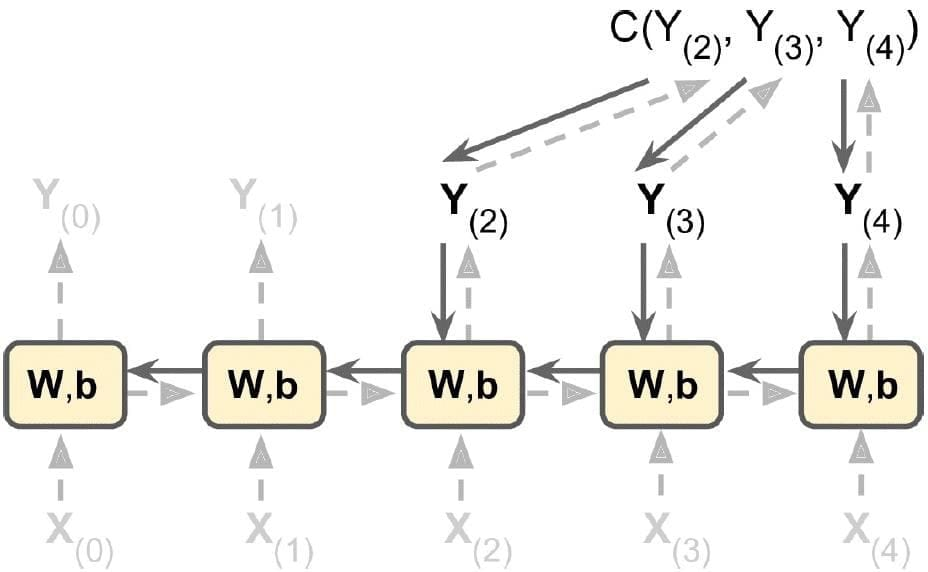## Training Recurrent Neural Networks

Recurrent Neural Networks are trained using the following steps:

• First, there is a forward pass through the unrolled network (dashed arrows).

• Then, the output sequence is evaluated using a cost function:

• C(Yt-min, Yt-min+1, …., Yt-max)

• The sequence starts with the t-min time step, and the outputs at some time steps may be ignored.

• Then the gradients of the unrolled network are propagated backward as shown by solid arrows.

• Finally, the model parameters are updated using the computed gradients.

Note that the gradients flow backward through all the outputs used by the cost function.

In the next section, let us learn how to train a sequence classifier.

## Training a Sequence Classifier

Let us understand how to train a Sequence Classifier in the following sections.

### MNIST Classifier

Let’s use RNNs to train an MNIST classifier.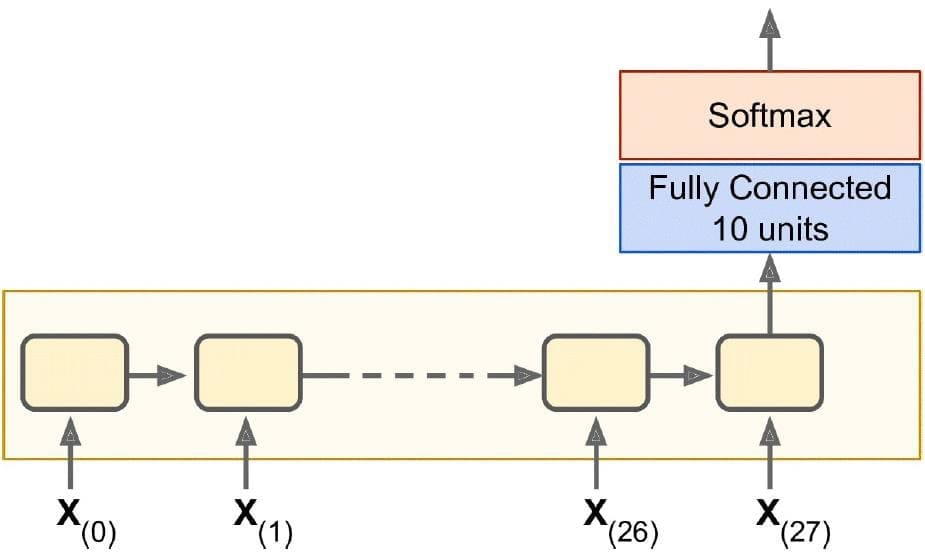Each handwritten digit image is 28 by 28 pixels, which is treated as 28 rows of 28 pixels each for purposes of RNN. You can use cells of 150 recurrent neurons.

Finally, there is a fully connected layer with 10 neurons (for 10 classes of digits), followed by a SoftMax layer for classification.

### Construction Phase

The construction phase of a sequence classifier is similar to a regular feedforward network, except the fact that the unrolled RNN replaces the hidden layers.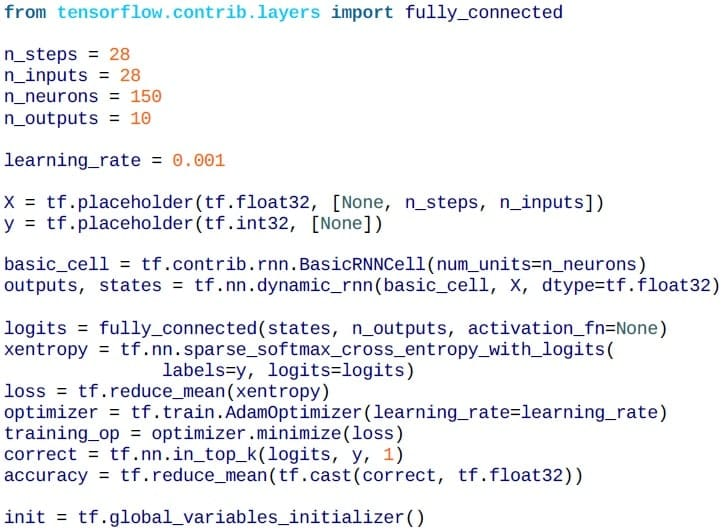Notice that the fully connected final layer is connected to the final state tensor states, which contains the 28th or the final output.

### Reshaping Test Data

Now, let’s load MNIST data and reshape the test data to [batch_size, n_steps, n_inputs].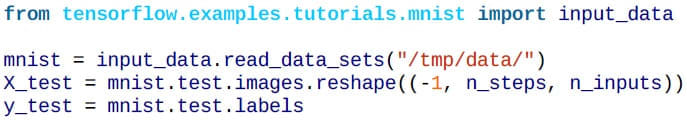### Execution Phase

The execution phase is similar to a regular neural network, except that you reshape each training batch before feeding it to the network.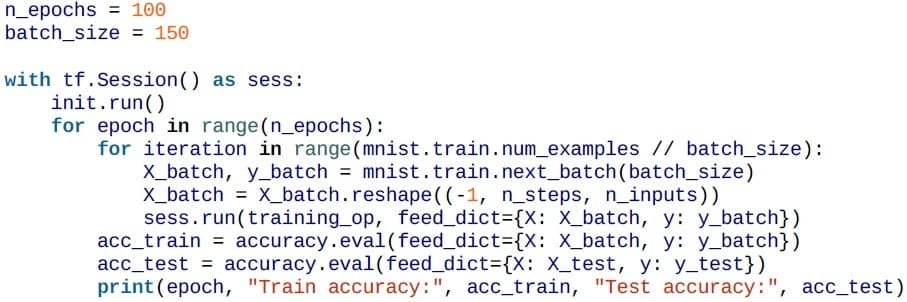### Output

The output is: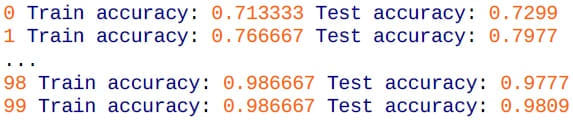The output demonstrates an accuracy of 98%.

You can tune it further by adjusting the hyperparameters, adding more epochs, or using He initialization or regularization (for example, dropout).

Let us understand time series predictions in the next section.

## Time Series Predictions

You can train an RNN to predict time series data such as stock prices, house values, air temperature, or even brain wave patterns.

Let’s develop a time series RNN where each training instance is a randomly selected sequence of 20 consecutive values. The target sequence is the same data shifted one step forward in time.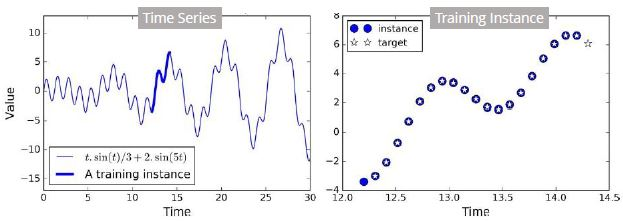Training

Let’s create the RNN:

The number of time steps is 20 since there are 20 points in each input sample. Each input has only one feature.

The target is also a sequence of 20 values, with stock prices shifted one step into the future. In practice, for a model like stock market prediction, you might have more than one feature. For example, each input might have not only stock price but also price of competing stocks, analyst’s ratings, the overall market cap of the entire market, etc.

At each time step, there is an output vector of size 100. But you want only one output value at each step. The simplest solution for this is an OutputProjectionWrapper. This adds a fully connected layer for each time step output (linear neurons without any activation function).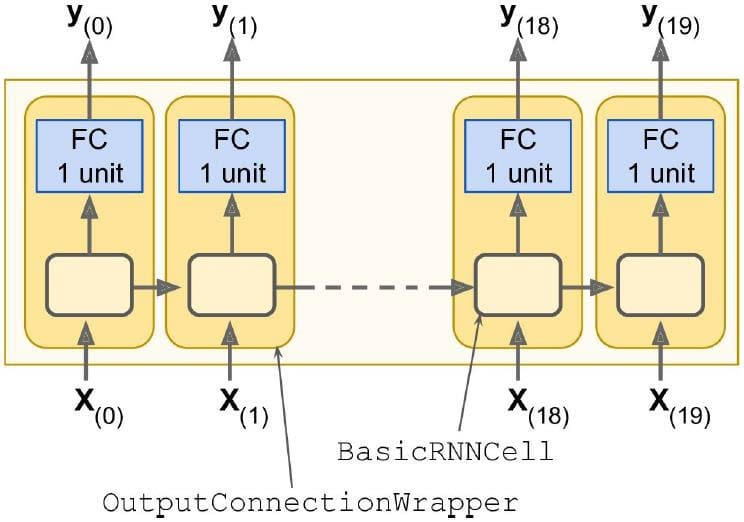The hidden states are not impacted. All the fully connected layers share the same trainable weights and bias terms.

The code to apply an OutputProjectionWrapper: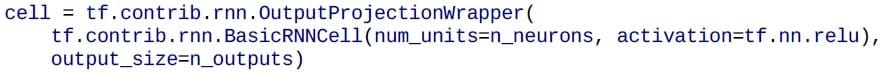You define the cost function using MSE and AdamOptimizer: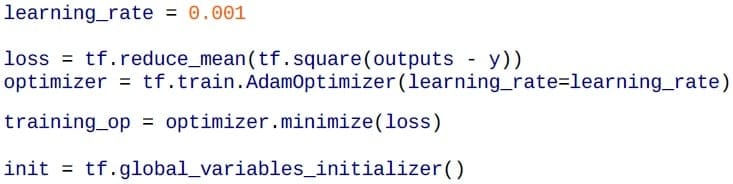Execution

Then comes the execution phase of the time series: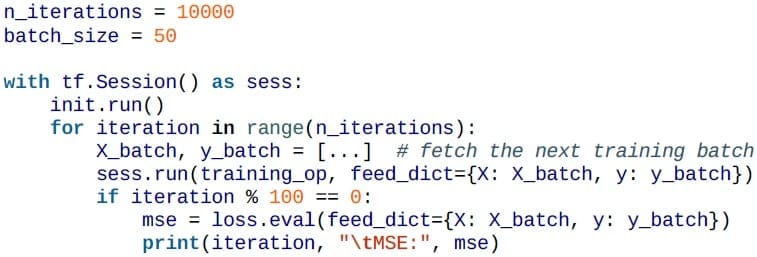Output

The output of the program is as follows: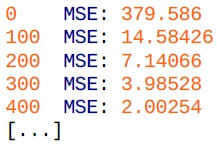Predictions

Once the model is trained, one can make predictions: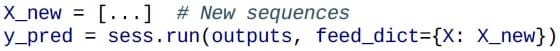Testing the Model

The figure given below shows the predicted sequence for the instance after 1000 training iterations: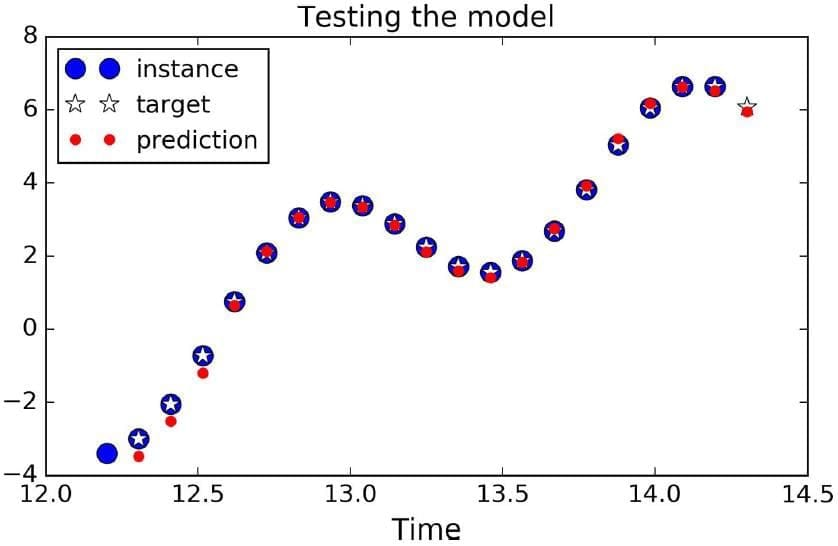## Deep Recurrent Neural Networks

The model explained in prior sections was pertaining to one layered RNN. It is possible to stack individual RNN layers to create a multi-layered RNN or Deep RNN. Deep RNNs can be created by stacking many layers of cells.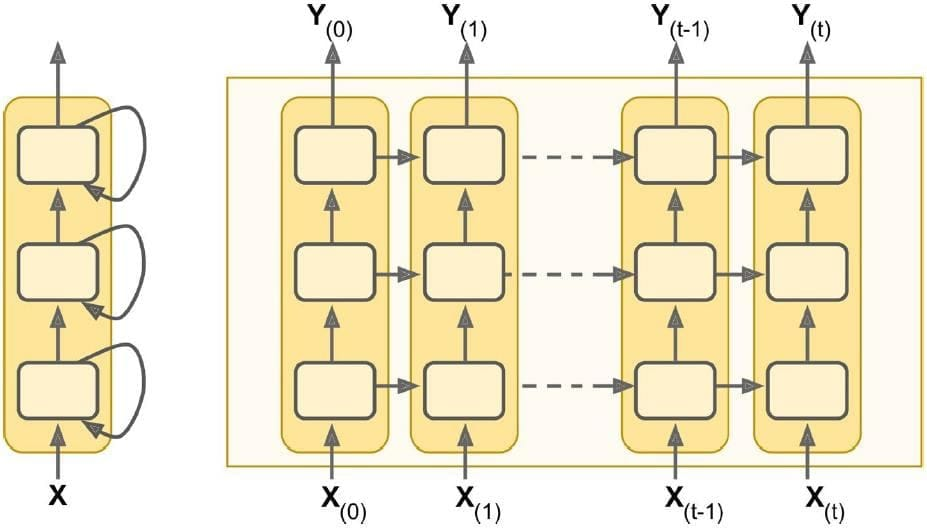In the next section, let us focus on the three-layered deep RNN.

## Three Layered Deep Recurrent Neural Networks

In TensorFlow, one can use MultiRNNCell, which is created by stacking several BasicRNNCells. The stacked cells can be of different kinds. Let’s create a three-layered deep RNN.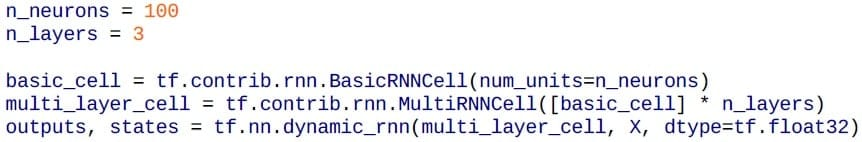For the code given above, states will be a tuple containing one tensor per layer, with each tensor representing the final state of the cell.

The shape of each state tensor will be [batch_size, n_neurons].

If you set the parameter state_is_tuple = False while creating a MultiRNNCell, then states becomes a single tensor, containing states of all three layers and with shape [batch_size, n_layers * n_neurons].

In the next section, let us focus on the vanishing gradient problem.

The vanishing or exploding gradient issue crops up with Deep RNNs. The techniques that can be used to prevent this are:

• Good parameter initialization

• Non Saturating activation functions (e.g., ReLU)

• Batch Normalization

• Faster optimizers

However, Deep RNN training is slow, even with as many as 100 input sequences. One solution is truncated backpropagation over time. To do this, you can truncate the input sequence. But the issue with this is that long sequences cannot be learned.

Another issue with RNNs is that memory of initial inputs gradually gets diluted and finally lost in later time steps. In some cases, the initial memory has significant value. For example, the first few words of a movie review given by audience might hold the key to user sentiment, regardless of later wordings.

This is where LSTM (Long Short-term Memory) cells come to rescue.

In the next section, let us focus on the LSTM cells.

## LSTM Cells

LSTM cells manage long-and short-term memory by means of logic gates, which control what information gets passed forward and what gets dropped.

LSTMs are very successful in modeling long-term time series, long texts, audio recordings, etc. In TensorFlow, LSTM cell can be created via the use of BasicLSTMCell:

lstm_cell = tf.contrib.rnn.BasicLSTMCell(num_units=n_neurons)

LSTM cells perform much better, converge faster, and learn long-term dependencies. An LSTM cell manages two state vectors instead of just one.

In the next section, let us discuss the state of LSTM cells.

## State of LSTM Cells

An LSTM cell has two states:

• c(t)-long-term state

• h(t)-short-term state

The long-term state passes through the forget gate to forget some memories and input gate to allow some memories. The long-term state is then passed through the gate without transformation.

The long-term state is also taken through tan h operation. The result is filtered by the output gate to produce short-term state h(t). This is equal to the cell’s output for the time step y(t).

Let’s see how the gates work.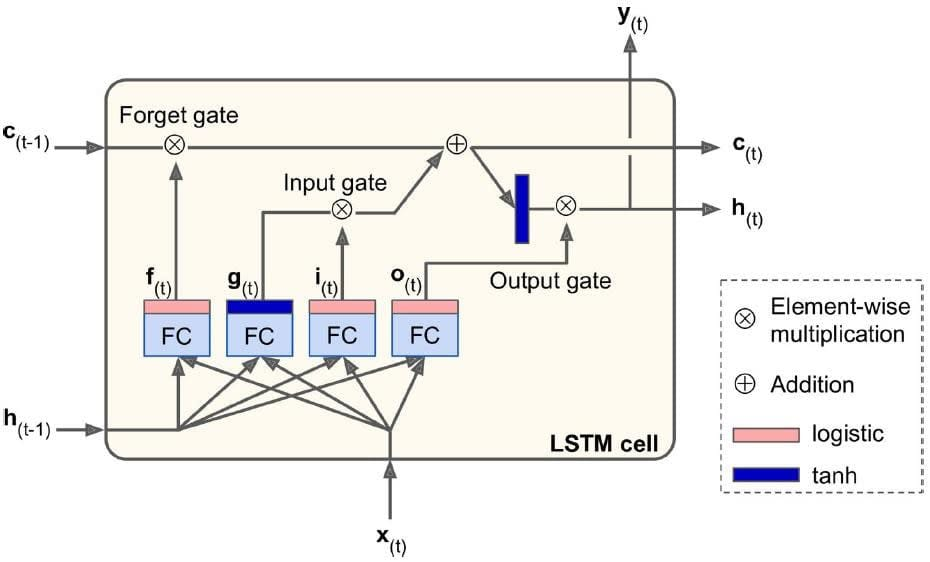The current input x(t)and previous short-term state h(t)are fed to four fully connected layers.

The main layer is the one producing output g(t). This layer is partially stored in the long-term state (controlled by the input gate).

The other three layers are gate controllers. They use the logistic activation function, so their output can be 0 or 1. If the output is 0, the corresponding gate is closed or else it is left open.

The output f(t) controls the forget gate, which decides what part of the long-term state is deleted.

The output i(t) controls the input gate, which decides what part of g(t) gets added to the long-term state.

The output o(t) controls the output gate, which decides what part of the long-term state can be stored in h(t) and y(t).

In the next section, let us understand the LSTM cell computations.

## LSTM Cell Computations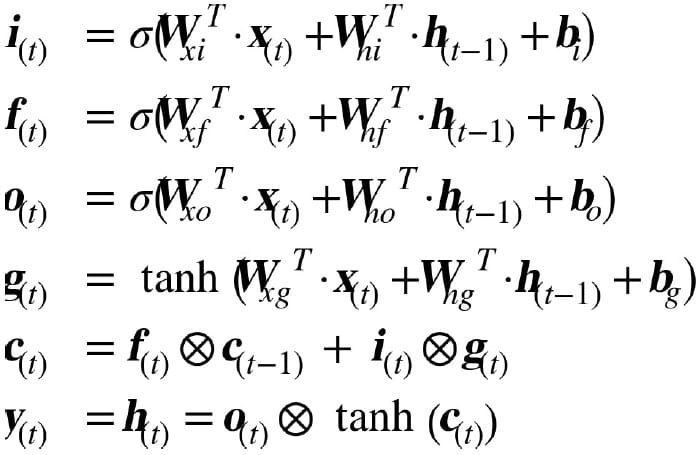Wxi, Wxf, Wxo, Wxg are the weight matrices of each of the four layers for their connection to the input vector x(t).

Whi , Whf, Who, Whg are the weight matrices of each of the four layers for their connection to the previous short-term state h(t-1).

bi, bf, bo, bg are the bias terms for each of the four layers. Note that TensorFlow initializes bf to a vector full of 1s instead of 0s. This prevents forgetting everything at the beginning of training.

Let us focus on the Word Embeddings in the next section.

Willing to take up a course in Deep Learning? Check out our Deep Learning Course Preview now!

## Word Embedding

RNNs are very useful for language modeling or language translation. For a vocabulary that is 50000 words strong, each nth word can be represented by a sparse vector 50000 long, with all 0 values except one in the nth position. This is very inefficient for large word set.

One solution is to use Word Embeddings. Here, one creates a word map with the proximity of like or similar words. A sentence like “I like milk” can be easily be replaced by “I like water” as milk and water can be closely placed in the word map.

An embedding is a very small but dense vector with 150 dimensions. To train word embeddings, initially, it is seeded randomly with words. During training with a neural network, the similar words end up huddling close to each other.

The results are amazing. For example, words might get placed on dimensions like verb/noun, singular/plural, etc.

Let’s see the code to create word embeddings.

Let’s create the embedding and initialize it randomly: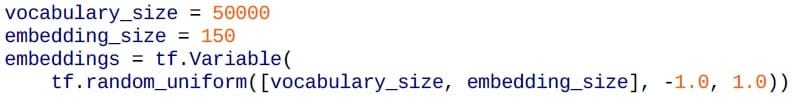Assume a sentence “I drink milk.” You wish to get its embeddings.

First, you need to break this into a list of known words.

Unknown words may be replaced by the token “[UNK]”, numerical values may be replaced by “[NUM]”, URLs by “[URL]”, etc.

Look up integer identifier for each word (0 to 49,999) in the dictionary.

The embedding_lookup function will get the corresponding embeddings for this sentence.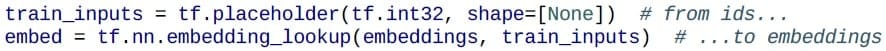One can train one’s own embeddings or use previously trained embeddings (much like pre-trained layers of a neural net).

In the next section, let us learn about the machine translation model.

## Machine Translation Model

An Encoder-Decoder RNN can translate across languages; for example, English to French. Notice that target output is also fed to the Decoder but pushed back by one step.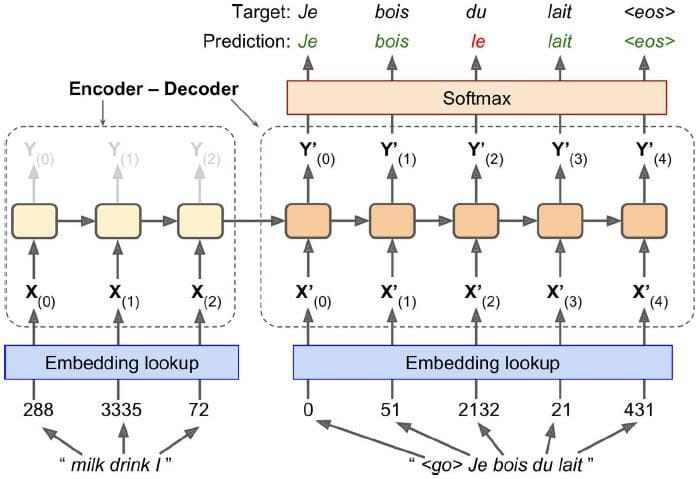<go> is a token for the beginning of a sentence and <eos> is for the end of a sentence.

Also, input English sentence is reversed first so that Decoder starts working with first word “I” first.

The Embeddings are what is fed to both Encoder and Decoder.

The Decoder outputs a score for each word in the output vocabulary, and Softmax converts this into a probability. For each step in the Decoder, the word with the highest probability is output.

Note that during production (inference time), the target sentence will not be available to feed to the Decoder.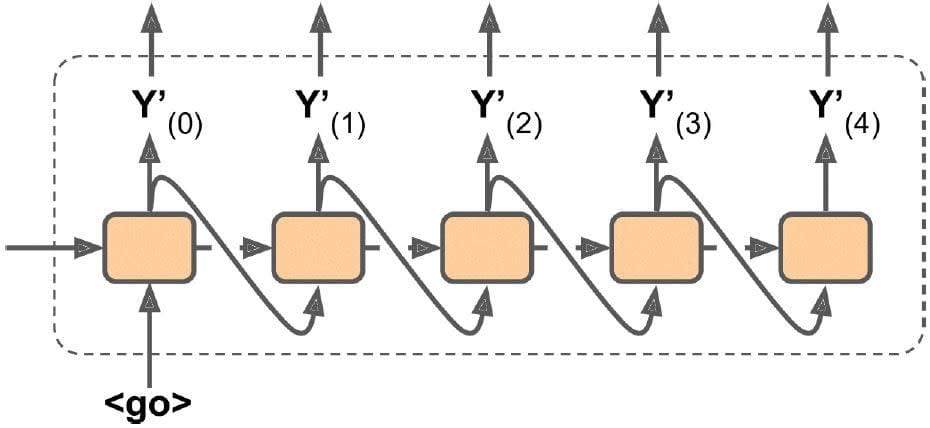Here, you simply feed the output word from previous time step to the current time step. (This will require an embedding lookup that is not shown in the figure).

## Summary

Let us summarise what we have learned in this lesson:

• RNNs are useful for processing data that is sequential in nature.

• An RNN involves a recurrent neuron, where the output of the neuron is fed back as an input to itself.

• RNNs can exist in various configurations.

• TensorFlow can be used to implement basic RNNs or dynamic RNNs.

• RNNs are trained via backpropagation through time or BPTT, similar to backpropagation logic of classic ANNs.

• RNNs can be used to predict time-series data like stock prices.

• LSTMs are a special form of RNN that use gate controllers to selectively preserve or delete the memory of data.

• Word Embeddings simplify processing of large word dictionaries, allowing development of sophisticated NLP applications.

• An Encoder-Decoder model of RNN can be used for language translation models.

## Conclusion

This concludes Recurrent Neural Networks. The next lesson focuses on the other forms of Deep Learning.

### Find our Deep Learning with Keras and TensorFlow Online Classroom training classes in top cities:

Name Date Place
Deep Learning with Keras and TensorFlow 25 Apr -17 May 2020, Weekend batch Your City View Details
Deep Learning with Keras and TensorFlow 16 May -7 Jun 2020, Weekend batch San Francisco View Details
Deep Learning with Keras and TensorFlow 22 May -13 Jun 2020, Weekdays batch Dallas View Details
Related Courses
Learner Reviews
Related Articles
• Disclaimer
• PMP, PMI, PMBOK, CAPM, PgMP, PfMP, ACP, PBA, RMP, SP, and OPM3 are registered marks of the Project Management Institute, Inc.

Name*
Email*
Phone Number*